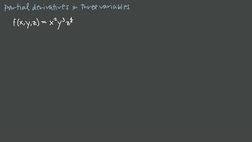PLAY PREVIEW

### Segments in this Video

#### Finding Partial Derivatives of Three Variable Functions(00:60)

FREE PREVIEW

To take the partial derivative of a three variable function, you need to take one partial derivative with respect to each variable. When taking a partial derivative, remember to treat other variables as if they were constants.

#### Calculate the First Order Partial Derivative(02:13)

By taking the first order partial derivative with respect to x, we can see that the constants here, y and z, just end up as the coefficient in front of the x squared term.

#### Simplifying the Partial Derivative(02:13)

Once the function has been differentiated, simplify.

#### Credits(00:00)

Credits

For additional digital leasing and purchase options contact a media consultant at 800-257-5126
(press option 3) or sales@films.com.

# Partial derivatives in three or more variables

Part of the Series : Integral Calc: Calculus 3
 3-Year Streaming Price: \$49.95

Share

### Description

This video tutorial works through math problems/equations that address topics in Calculus 3, Partial Derivatives. This specific tutorial addresses Partial derivatives in three or more variables.

Length: 6 minutes

Item#: FMK275741Closed Captioned